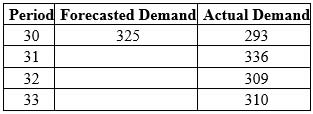# What is the forecast for period 34 if a simple three-period moving average is used?

The question below is based on the following information: What is the forecast for period 34
if a simple three-period moving average is used?A.
325

B.
318

C.
312

D.
313## One Comment on “What is the forecast for period 34 if a simple three-period moving average is used?”

1.networkmanagers says:

I choose B

0

0